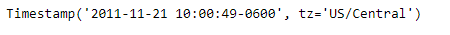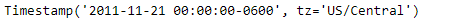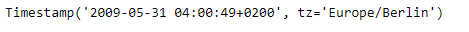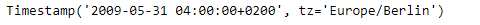# Python | Pandas Timestamp.floor

Python is a great language for doing data analysis, primarily because of the fantastic ecosystem of data-centric python packages. Pandas is one of those packages and makes importing and analyzing data much easier.

Pandas` Timestamp.floor()` function return a new Timestamp floored to this resolution. The function takes the desired time series frequency as an input.

Syntax : Timestamp.floor()

Parameters :
freq : a freq string indicating the flooring resolution

Return : Timestamp

Example #1: Use `Timestamp.floor()` function to floor the given Timestamp object to Daily time series frequency.

 `# importing pandas as pd ` `import` `pandas as pd ` ` `  `# Create the Timestamp object ` `ts ``=` `pd.Timestamp(year ``=` `2011``,  month ``=` `11``, day ``=` `21``, ` `           ``hour ``=` `10``, second ``=` `49``, tz ``=` `'US/Central'``) ` ` `  `# Print the Timestamp object ` `print``(ts) `

Output :Now we will use the `Timestamp.floor()` function to floor the ts object to Daily frequency.

 `# floor the given object to daily frequency ` `ts.floor(freq ``=``'D'``) `

Output :As we can see in the output, the `Timestamp.floor()` function has floored the time series frequency of the given Timestamp object to the input frequency.

Example #2: Use `Timestamp.floor()` function to floor the given Timestamp object to minutely time series frequency.

 `# importing pandas as pd ` `import` `pandas as pd ` ` `  `# Create the Timestamp object ` `ts ``=` `pd.Timestamp(year ``=` `2009``,  month ``=` `5``, day ``=` `31``,  ` `       ``hour ``=` `4``, second ``=` `49``, tz ``=` `'Europe/Berlin'``) ` ` `  `# Print the Timestamp object ` `print``(ts) `

Output :Now we will use the `Timestamp.floor()` function to floor the ts object to minutely frequency.

 `# floor the given object to minutely frequency ` `ts.floor(freq ``=``'T'``) `

Output :As we can see in the output, the `Timestamp.floor()` function has floored the time series frequency of the given Timestamp object to the input frequency.

My Personal Notes arrow_drop_upCheck out this Author's contributed articles.

If you like GeeksforGeeks and would like to contribute, you can also write an article using contribute.geeksforgeeks.org or mail your article to contribute@geeksforgeeks.org. See your article appearing on the GeeksforGeeks main page and help other Geeks.

Please Improve this article if you find anything incorrect by clicking on the "Improve Article" button below.

Article Tags :

Be the First to upvote.

Please write to us at contribute@geeksforgeeks.org to report any issue with the above content.